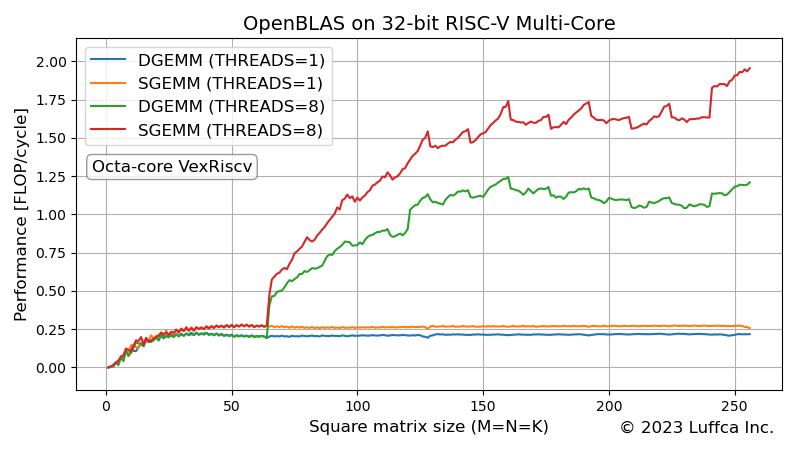# OpenBLAS on 32-bit RISC-V Multi-CoreWe made OpenBLAS compatible with 32-bit RISC-V and evaluated the performance of GEMM (GEneral Matrix-to-matrix Multiply) using an FPGA board with octa-core 32-bit RISC-V SoC.

## OpenBLAS

The Introduction of README.md of OpenBLAS has the following description.

OpenBLAS is an optimized BLAS (Basic Linear Algebra Subprograms) library based on GotoBLAS2 1.13 BSD version.

As for RISC-V support, TargetList.txt of the latest release v0.3.21 has `RISCV64_GENERIC` for 64-bit RISC-V and `C910V` for XuanTie C910 that supports RISC-V Vector (0.7.1).

## OpenBLAS for RV32GC

VexRiscv supports RISC-V single-precision floating point extension F, and also supports double-precision floating point extension D, which is rare for 32-bit RISC-V. So we built OpenBLAS for VexRiscv with RV32IMAFDC (RV32GC) support.

Also, to enable OpenMP, build with `USE_OPENMP=1` option.

## GEMM on Octa-Core VexRiscv

We evaluated the performance of DGEMM (double precision GEMM) and SGEMM (single precision GEMM) by combining the Nexys Video FPGA board with octa-core VexRiscv SoC and the Linux environment created using Buildroot. The SoC is introduced in the article OpenMP on FPGA with RISC-V Multi-Core Processor.

The following shows the console output when running SGEMM with 8 threads. By setting the environment variable `OPENBLAS_LOOPS` to 10, the average performance of 10 times is calculated.

```root@buildroot:/home# export OPENBLAS_LOOPS=10
root@buildroot:/home# ./sgemm.goto 1 256
From :   1  To : 256 Step=1 : Transa=N : Transb=N
SIZE                   Flops             Time
M=   1, N=   1, K=   1 :        0.04 MFlops   0.000468 sec
M=   2, N=   2, K=   2 :        0.60 MFlops   0.000268 sec
M=   3, N=   3, K=   3 :        1.55 MFlops   0.000349 sec
...
M= 254, N= 254, K= 254 :      194.70 MFlops   1.683302 sec
M= 255, N= 255, K= 255 :      193.38 MFlops   1.714861 sec
M= 256, N= 256, K= 256 :      195.52 MFlops   1.716203 sec
```

The featured image shows the performance (FLOP/cycle) of DGEMM and SGEMM. Since the operating frequency of VexRiscv is 100MHz, 1FLOP/cycle corresponds to 100MFLOPS.

## Summary

We made OpenBLAS compatible with 32-bit RISC-V and evaluated the performance of GEMM using the Nexys Video FPGA board with octa-core VexRiscv SoC.

Previous article

Next article